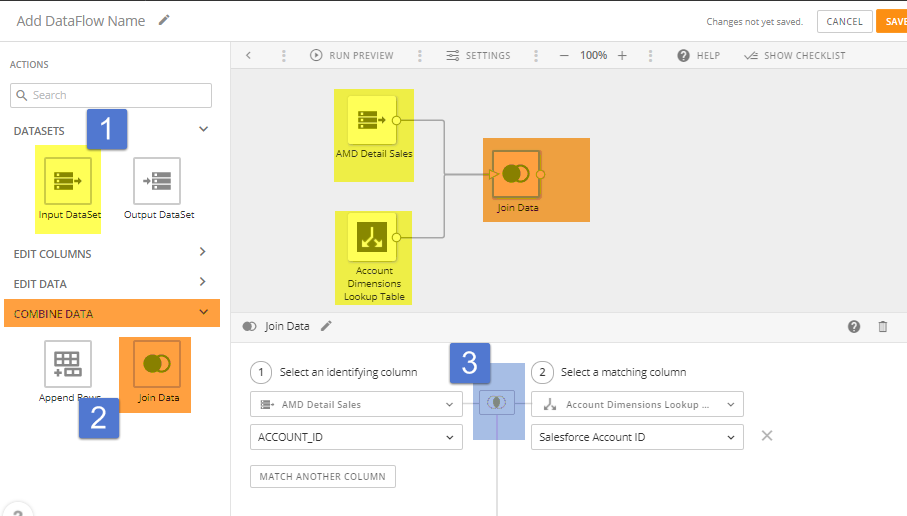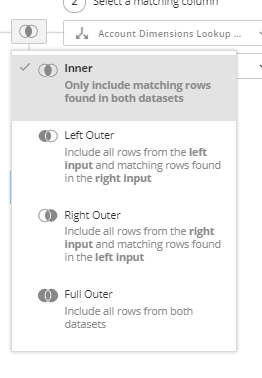# Vlookups in DOMO. How do we use Vlookup functionality in DOMO in Beast Mode ?

• Can you give us an example of a situation for your particular vlookup needs? The answer might be different depending on what you are looking for.

It might be a dataflow that you would need to use, it might be a case statement in a beast mode, or something else entirely.

• as @AS mentioned, there are many different ways to do this.  Your particular use may make one way better than another.  However, in general, Domo accomplishes this by using a "join".  The join is a tool that looks at one dataset and matches a column (or field) with a field in another data set.  The field that you join on would be the "search" term from Excel.  I will show you an example using ETL:You will need to pick 2 data sets, or tables, to join (1).  You then select the "Join Data" action (2).  You connect the two input data sets to the Join data tile.  In the settings for the Join data tile you need to select your identifying column.  It will then join all of the data from each data set that are related to each unique identifying column.  You can select 4 different kinds of joins (3).• Example of Vlookup in the scenario Im trying to execute is : I have some G/L accounts, for which have perfomed calculations like age in Months. Have a Vlookup that makes certain G/L account as OLD /NEW for which I am using Vlookup.

So I need to use the calculation of Age in Months in Beast Mode when I compare it with the VLookup data.

I understand, that Vlookup in SQL can be performed via joins, because the table in which will perform Vlookup will remain intact. an dwill get the errors for null values.

So, I just need to implement the comparison of Age months and this calculation should compare it with the Vlookup data and determine if its old/New G/L account.

And the final visulations will have values of OLD/NEW GL and Age in Months.

• if the `OLD/NEW` field is only determined by the `Age in Months` field, then you can do this via a beastmode case statement:

CASE WHEN `Age in Months` >= 24 THEN 'OLD' ELSE 'NEW' END

name the beastmode whatever you would like the field to be called (i.e.`Old/New GL`)

You can enter whatever number you want to define as old or new.  I used 24 months to denote an old account.

• Thanks for the response, but Old/New G/L is dependant on the Vlookup. How am I suppose to put that together?

Old/NEw G/L

:if (period_diff(date_format(CURDATE()+15, '%Y%m'),date_format(`posting Date`, '%Y%m'))>`G/L`,`GL`:`Current`,2,0),1,0)).

Here GL:current is the Vlookup table.

Also, I dragged the calculation of Age in months from measures in this field, which is this : (period_diff(date_format(CURDATE()+15, '%Y%m'),date_format(`posting Date`, '%Y%m')).

Not sure, if this is the safe bet ?

• Could you provide a sample of your data and what you are wanting as a result?  I'm not sure I am fully understanding what your data looks like and what output you are looking for.

• Hi,

I am looking how to traverse OLD G/L value from V look up instead of hard coding it. So V-lookup has values like

 GL Current 13000220 3 13000230 3 14200300 12 14200301 12 14200500 12 25800700 12 25900100 12

So, in the code for now I have hard coded in formulae to find if the A/C is old/ current like this :

CASE
WHEN period_diff(date_format(CURDATE()+15, '%Y%m'),date_format(`Posting Date in the Document`, '%Y%m'))>3 THEN  'OLD'
WHEN  period_diff(date_format(CURDATE()+15, '%Y%m'),date_format(`Posting Date in the Document`, '%Y%m'))>12 THEN 'OLD'
else 'Current'
END       .

As it was easy to hard code just 2- different values in V lookup to drive the Old account/ current.

Eg: G/L-13000220, if the Age in Months is >3 it will be considered as OLD else Current.

But 3 is just a variable which tend to change later. So is there a way/option of not hard coding it. And coming up with better solution.

100.PNG 13.8K